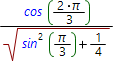# Simplify expression onlineThis calculator allows one to simplify math expression using symbolic arithmetics, i.e. not only numbers allowed as an inputs but also the variables (parameters) are accepted. The calculator tries to simplify expression specified. At present, calculator is able to simplify similar terms, calculate values of functions at some values of their arguments and many others.

Input you expression in the input expression field of calculator. To input the correct expression you may need to check math input rules. As soon as you input mathematicaly correct expression it will be shown above the expression input field to illustrate the expression input process. WARNING! If you input mathematically incorrect expression the error message with recomendations of correct input will be shown.

Simplify expressions calculatorExpression you want to simplify: